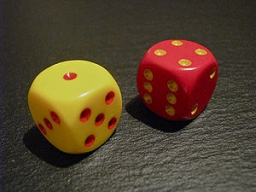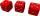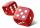# We roll

We roll two dice
A. - what is the probability that the sum of the falling numbers is at most 4
B. - is at least 10
C. - is divisible by 5?

a =  0.1667
b =  0.1667
c =  0.1944

### Step-by-step explanation:Did you find an error or inaccuracy? Feel free to write us. Thank you!Tips to related online calculators
Would you like to compute count of combinations?

## Related math problems and questions:

• Two diceWe roll two dice. What is the probability that the sum of the falling numbers is greater than 3?
• Three diceWhat is the probability that when we roll three dice, we roll the numbers 1,2,3?
• Fall sum or sameFind the probability that if you roll two dice, it will fall the sum of 10, or the same number will fall on both dice.
• Roll the diceWhat is the probability that if we roll the dice, a number less than 5 falls?
• Air draftThe numbers 1,2,3,4,5 are written on five tickets on the table. Air draft randomly shuffled the tickets and composed a 5-digit number from them. What is the probability that he passed: and, the largest possible number b, the smallest possible number c, a
• Sum 10What is the probability that two dice throw twice in a row will result the sum of 10?
• Six on diceWhat is the probability that when throwing two dice will fall at least one six?
• Divisible by fiveHow many different three-digit numbers divisible by five can we create from the digits 2, 4, 5? The digits can be repeated in the created number.
• ThrowWe throw 2 times with 2 dices. What is the probability that the first roll will fall more than sum of 9 and the second throw have sum 3 or does not have the sum 4?
• One threeWe throw two dice. What is the probability that max one three falls?
• How many 4How many four-digit numbers that are divisible by ten can be created from the numbers 3, 5, 7, 8, 9, 0 such no digits repeats?
• Three digits numberFrom the numbers 1, 2, 3, 4, 5 create three-digit numbers that digits not repeat and number is divisible by 2. How many numbers are there?
• Drawing from a hatWhen drawing numbers from a hat from 1 to 35, we select random given numbers. What is the probability that the drawn numbers will be divisible by 8 and 2?
• DiceWe throw 10 times a playing dice. What is the probability that the six will fall exactly 4 times?
• The diceWhat is the probability of events that if we throw a dice is rolled less than 6?
• Three-digit integersHow many three-digit natural numbers exist that do not contain zero and are divisible by five?
• Sum or productWhat is the probability that two dice fall will have the sum 7 or product 12?